Ch 5. Rigid Body General Motion Multimedia Engineering Dynamics Fixed Axis Rotation Plane Motion Velocities Zero Velocity Point Plane Motion Accelerations Multiple Gears Rot. Coord. Velocities Rot. Coord. Acceleration
 Chapter - Particle - 1. General Motion 2. Force & Accel. 3. Energy 4. Momentum - Rigid Body - 5. General Motion 6. Force & Accel. 7. Energy 8. Momentum 9. 3-D Motion 10. Vibrations Appendix Basic Math Units Basic Equations Sections Search eBooks Dynamics Fluids Math Mechanics Statics Thermodynamics Author(s): Kurt Gramoll ©Kurt GramollDYNAMICS - THEORY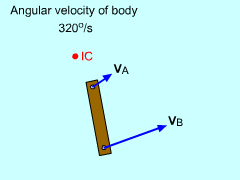Moving IC point

The "Instantaneous Center of Zero Velocity" or IC, is the point on a moving body that has zero velocity. The IC does not have to be on the actual body.

The IC may move as the body moves. If the IC is used as the reference point, the relative velocity equation becomes

vB = vA + vB/A

vB = 0 + vB/A

vB = ω rB/A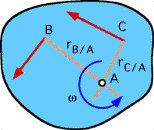Perpendicular Lines from Two Know
Velocity Vectors Locate the IC point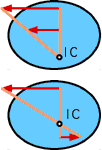Special Cases With
Parallel Velocity Vectors

This is the same relationship that was developed for fixed rotation of a rigid body. The only difference is IC may not be a fixed point, and needs to be found.

In general, the velocity direction and magnitude of one point and the velocity direction of another point on the same body need to be known to use the IC method.

In most cases, lines perpendicular to the two velocity vectors will intersect at the IC point as shown.

If the velocity vectors are parallel, then the distance from the IC to the velocity vectors is proportional, as shown in the figures.

If the velocity vectors are parallel and have the same magnitude and direction, then there is no IC and the body has no rotation.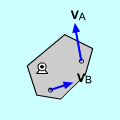Example 1 of IC point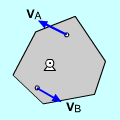Example 2 of IC point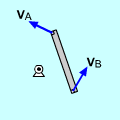Example 3 of IC point

Practice Homework and Test problems now available in the 'Eng Dynamics' mobile app
Includes over 400 problems with complete detailed solutions.
Available now at the Google Play Store and Apple App Store.